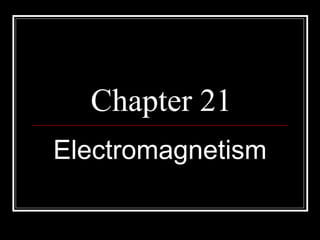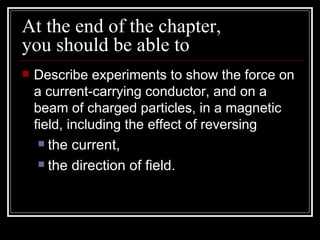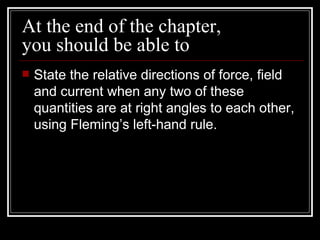Successfully reported this slideshow.

# Electromagnetism×

# Electromagnetism

Here you can find out more about magnetic fields due to a current carryin wire, Lorentz force and Fleming's left-hand rule.

Here you can find out more about magnetic fields due to a current carryin wire, Lorentz force and Fleming's left-hand rule.

### Electromagnetism

1. 1. Chapter 21 Electromagnetism
2. 2. At the end of the chapter, you should be able to  Describe experiments to show the force on a current-carrying conductor, and on a beam of charged particles, in a magnetic field, including the effect of reversing  the current,  the direction of field.
3. 3. At the end of the chapter, you should be able to  State the relative directions of force, field and current when any two of these quantities are at right angles to each other, using Fleming’s left-hand rule.
4. 4. At the end of the chapter, you should be able to  Explain how a current-carrying coil in a magnetic field experiences a turning effect and that the effect is increased by increasing  the number of turns on the coil,  the current.
5. 5. At the end of the chapter, you should be able to  Discuss how this turning effect is used in the action of an electric motor.  Describe the action of a split-ring commutator in a two-polt, single-coil motor and the effect of winding the coil on to a soft-iron cylinder.
6. 6. Magnetic Effect of a Current  Electric current produce a magnetic field even if the wire itself is not made of magnetic material.
7. 7. Magnetic Effect of a Current Magnetic field pattern due to a straight wire.  The direction of the magnetic field can be determined by the Right-hand rule. If you imagine that the conductor is held in the right hand, with the thumb pointing in the direction of the current flow, then the fingers curl the direction of the magnetic field
8. 8. Magnetic Effect of a Current Magnetic field pattern due to a straight wire.  The direction of the magnetic field can be determined by the Right-hand rule. current Current flowing out of paper
9. 9. Magnetic Effect of a Current Magnetic field pattern due to a straight wire.  The direction of the magnetic field can be determined by the Right-hand rule. current Current flowing into the paper
10. 10. Magnetic Effect of a Current Magnetic field pattern due to a flat coil
11. 11. Magnetic Effect of a Current Magnetic field pattern due to a flat coil  To increase the strength of the magnetic field at the centre of the flat coil. - Increase the current in the coil. - Increase the number of turns of the flat coil.
12. 12. Magnetic Effect of a Current Magnetic field pattern of a solenoid
13. 13. Magnetic Effect of a Current Magnetic field pattern of a solenoid  To increase the strength of the magnetic field of a solenoid. - Increase the current in the solenoid. - Increase the number of turns per unit length of the solenoid. - Using a soft-iron core within the solenoid.
14. 14. Quiz  Describe an experiment that will allow you to observe the magnetic field patterns produced by a current-carrying straight wire.
15. 15. Applications of a Magnetic Effect of Current  circuit breaker A ______________ is used to protect the appliance from excessive current flow.
16. 16. Applications of a Magnetic Effect of Current  current When a larger _______ flows through the circuit breaker, the magnetic field produced stronger by the solenoid becomes ___________.  The solenoid becomes a ______________ strong electromagnet. attract It is now able to ___________ the latch and the spring decompresses. The spring pushes the safety bar out of the interrupt switches off point and ___________ the circuit.
17. 17. Applications of a Magnetic Effect of Current  When the reset button is pushed, the spring compresses and the safety bar returns to close _______ the interrupt point. The spring stays compressed due to the soft iron latch ___________ and current can flow through the circuit breaker again.
18. 18. Applications of a Magnetic Effect of Current  A circuit breaker is not suitable for use with high voltage electricity because the electric flow current can _______ across the small opening in the interrupt point when the potential difference is sufficiently high ___________.
19. 19. Quiz  State the effect of a larger current on the magnetic force between the solenoid and the soft iron latch in a circuit breaker. The magnetic force will be stronger.
20. 20. Force on a Current-Carrying Conductor in a Magnetic Field Investigation of the force on a current-carrying conductor in a magnetic field (Lorentz Force) (Textbook, Page 422)
21. 21. Force on a Current-Carrying Conductor in a Magnetic Field  Apparatus  Stiff wire, string permanent magnets, 9V d.c. power supply.
22. 22. Force on a Current-Carrying Conductor in a Magnetic Field  Procedures:  Bend a stiff wire ABCD into the shape of a swing as shown in the figure.
23. 23. Force on a Current-Carrying Conductor in a Magnetic Field  Procedures:  Place the magnet over the wire BC as shown.
24. 24. Force on a Current-Carrying Conductor in a Magnetic Field  Procedures:  Switch on the current. Observe the direction in which the wire is swung.
25. 25. Force on a Current-Carrying Conductor in a Magnetic Field  Procedures:  Reverse the direction of the current by switching the polarity of the dry cell. In which direction is the swing flung now?
26. 26. Force on a Current-Carrying Conductor in a Magnetic Field  Observations:  With the current flowing in the direction A --> B --> C --> D, the wire is observed to swing outwards from the magnet.  If the current is reversed, the swing of the wire will be reversed (inwards)
27. 27. Force on a Current-Carrying Conductor in a Magnetic Field  In conclusion to the experiment, we can say that  A force acts on the current-carrying wire when placed in a magnetic field.  The force acts at right angle to both the current direction and the direction of the magnetic field.
28. 28. Force on a Current-Carrying Conductor in a Magnetic Field  In conclusion to the experiment, we can say that  When the direction of current (or magnetic field) is reversed, the direction of the force on the wire is also reversed.
29. 29. Fleming’s Left-hand Rule  The direction of the force can be deduced by using this rule. Motion of force  thuMb - direction ofMotion of the wire.
30. 30. Fleming’s Left-hand Rule  The direction of the force can be deduced by using this rule. Motion of force Magnetic Field (N-S direction)  Forefinger - direction of magnetic Field
31. 31. Fleming’s Left-hand Rule  The direction of the force can be deduced by using this rule. Motion of force Magnetic Field Current  SeCond finger - direction of Current (Conventional current)
32. 32. Force on a Current-Carrying Conductor in a Magnetic Field  To explain the force exerted on the wire, we S need to consider the combined magnetic fields due to the current flowing through the straight wire and the magnet. N
33. 33. 22.1 Force on a Current-Carrying Conductor in a Magnetic Field  The wire moves because the magnetic field of the permanent magnets reacts with the magnetic field of the current in the wire. S N
34. 34. 22.1 Force on a Current-Carrying Conductor in a Magnetic Field  The two fields acting in the same direction combine to give a stronger field, but the two field opposing each other combine to give a weaker field. weaker field stronger field
35. 35. 22.1 Force on a Current-Carrying Conductor in a Magnetic Field  Hence the unbalanced fields on both sides produces a force that exerts on the wire. weaker field stronger field
36. 36. Force on a Moving Charge in a Magnetic Field  Fleming’s left-hand rule can be applied to all moving charges. Force Magnetic Field Current (conventional current)  Note that the conventional current (flow of positive charges) travels in an opposite direction to that of the electron flow.
37. 37. Moving-coil loudspeaker  It consists a movable coil attached to the large cone.
38. 38. Moving-coil loudspeaker  The cone fits loosely over the centre of a cylindrical permanent magnet so that the coil is in a strong magnetic field.
39. 39. Moving-coil loudspeaker  The wire of the coil is at right angles to the field.
40. 40. Moving-coil loudspeaker S  If the current flows in the direction shown about, the coil will move out of the page.
41. 41. Moving-coil loudspeaker S  If the direction of the current reverses, the coil will move in the opposite direction (into the page).
42. 42. Simple Circuit Breaker
43. 43. Simple Circuit Breaker • When the live wire carries the usual operating current the electromagnet is not strong enough to separate the contacts.
44. 44. Simple Circuit Breaker • If something goes wrong with the appliance and a large current flows the electromagnet will exert a strong magnetic force to separate the contacts and break the circuit. The spring then keeps the contacts apart.
45. 45. Simple Circuit Breaker • After the fault is repaired, the contacts can then be pushed back together by lifting a switch on the outside of the circuit breaker.